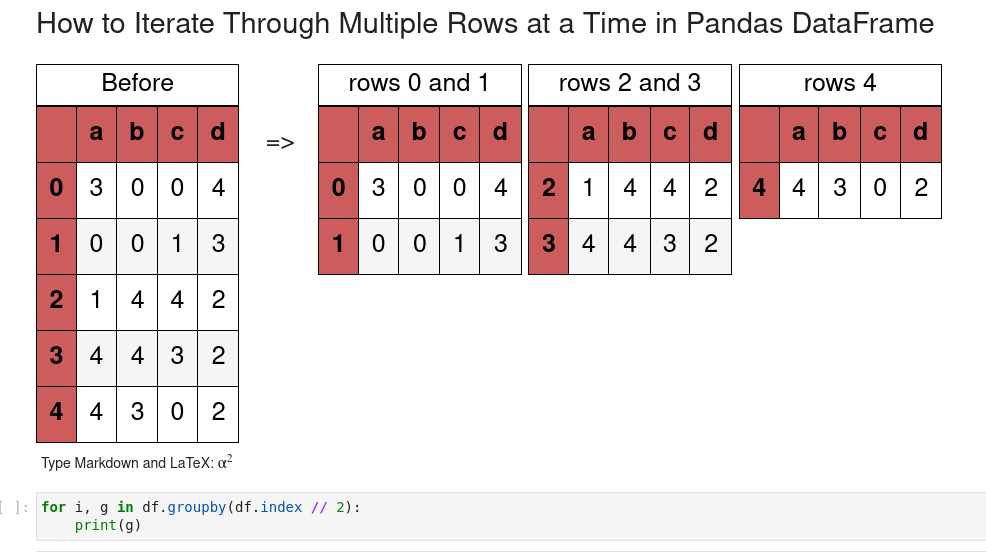In this short guide, I'll show you how to iterate simultaneously through 2 and more rows in Pandas DataFrame. So at the end you will get several rows into a single iteration of the Python loop.

If you like to know more about more efficient way to iterate please check: How to Iterate Over Rows in Pandas DataFrame

## Setup

Let's create sample DataFrame to demonstrate iteration over multiple rows at once in Pandas:

``````import numpy as np
import pandas as pd
import string

string.ascii_lowercase

n = 5
m = 4

cols = string.ascii_lowercase[:m]

df = pd.DataFrame(np.random.randint(0, n,size=(n , m)), columns=list(cols))
``````

Data will looks like:

a b c d
0 1 1 2 2
1 3 2 1 1
2 2 2 3 4
3 0 2 3 2
4 0 4 3 4

## Step 1: Iterate over 2 rows - RangeIndex

The most common example is to iterate over the default RangeIndex. To check if a DataFrame has `RangeIndex` or not we can use:

``````df.index
``````

If the result is something like:

``````RangeIndex(start=0, stop=5, step=1)
``````

Then we can use this method:

``````for i, g in df.groupby(df.index // 2):
print(g)
print('_' * 15)
``````

This will give us:

``````   a  b  c  d
0  1  1  2  2
1  3  2  1  1
_______________
a  b  c  d
2  2  2  3  4
3  0  2  3  2
_______________
a  b  c  d
4  0  4  3  4
_______________
``````

To access the values inside the loop we can use:

• row 1 - `g.values`
• row 2 - `g.values`
So we can see that the solution works fine even for an odd number of rows in DataFrame.

How does it work?

``````df.index // 2
``````

will do mod on 2 resulting in:

``````Int64Index([0, 0, 1, 1, 2], dtype='int64')
``````

Then we will group by the result `df.groupby(df.index // 2)`## Step 2: Iterate over n rows at once

So to iterate through n rows we need to change n in: `for i, g in df.groupby(df.index // n)`:

``````for i, g in df.groupby(df.index // 3):
print(g)
print('_' * 15)
``````

result:

``````   a  b  c  d
0  1  1  2  2
1  3  2  1  1
2  2  2  3  4
_______________
a  b  c  d
3  0  2  3  2
4  0  4  3  4
_______________
``````

## Step 3: Iterate over Index

A generic solution for DataFrame with non numeric index we can use numpy to split the index into groups like:

``````[0, 0, 1, 1, 2]
``````

To do so we use method `np.arrange` providing the length of the DataFrame:

``````for i, g in df.groupby(np.arange(df.shape) // 2):
print (g)
``````

result:

``````array([0, 0, 1, 1, 2])
``````

## Step 4: Iterate with iterrows + zip

Finally we can use `df.iterrows()` and `zip()` to iterate over multiple rows at once.

The limitation of this method is that it doesn't work for an odd number of rows (last row is skipped). Also is not good for iterating over n rows.

So combination of `df.iterrows()` and `zip()` to loop over 2 rows at the same time:

``````t = df.iterrows()
for (i, r1), (j, r2) in zip(t, t):
print(r1.values, r2.values)
``````

result:

``````[1 1 2 2] [3 2 1 1]
[2 2 3 4] [0 2 3 2]
``````

## Conclusion

We saw how to loop over two and more rows at once in Pandas DataFrame. We covered the case of Index vs RangeIndex.

Finally we saw an alternative way by combining `df.iterrows()` and `zip()` and the limitation of it.